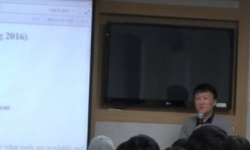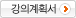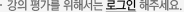## 주메뉴

### 경제수학

• 경희대학교
• 전택승• 주제분류
사회과학 >경영ㆍ경제 >경제학
• 강의학기
2016년 1학기
• 조회수
15,915
•
강의계획서본 경제수학 강의에 경제 분석의 기초가 되는 수학적인 기반을 다지는데 초점을 둡니다. 따라서 형식적인 이론의 나열보다는 다양한 사례를 통해 공부하여, 이 강의 이후에는 이론을 응용할 수 있는 능력을 가질 수 있게 하는 것이 수업의 목표입니다. The purpose of this module is to learn how to use mathematics to solve economic and social problems. This course develops the basic mathematical tools required for the advanced study of economics. Its purpose is to introduce and practice the application of mathematical methods and concepts to prepare students for an in-depth study of econometrics, microeconomics and macroeconomics.

#### 차시별 강의강의계획서1.Linear Equations and Matrix Linear Equations and MatrixLinear Equations and Matrix Syllabus2.Elimination EliminationElimination inverse matrixVector space - part 1 vector space 13.Vector space - part 1 201603164.Vector space - part II 20160328Vector space - part II vector space - part II5.Linear independence, span, basis, and dimension 20160330Linear independence, span, basis, and dimension Linear independence, span, basis, and dimension6.Solving the system solving the system of equations7.Determinant- part1 determinant8.Determinant - part2 Determinant9.Inverse, Ax=b, and Determinant Inverse, Ax=b, and Determinant10.Midterm Solutions midtermEigenvalues and Eigenvectors Eigenvalues and Eigenvectors11.Eigenvalues and Eigenvectors 20160427Eigenvalues and Eigenvectors - part 2 Eigenvalue and Eigenvector - part 212.Eigenvalues and Eigenvectors - part 2 20160504Differentiation - part 1 Differentiation - part 113.Differentiation - part 1 20160511Differentiation - part 2 Differentiation part 214.Differentiation - part 2 20160525Differentiation - part 3 Differentiation part 315.Differentiation - part 3 20160530Optimization - part 1 20160601Optimization - part 1 optimization part 1Optimization - part 2 optimization - part 2Optimization - part 2 20160608Practice problems for Final practice problemsPractice problems for Final 20160613#### 연관 자료#### 사용자 의견#### 이용방법

• 플래쉬 유형 강의 이용시 필요한 프로그램 [바로가기]
문서 자료 이용시 필요한 프로그램 [바로가기] 인터넷 익스플로러-도구-호환성보기설정-khu.ac.kr

※ 강의별로 교수님의 사정에 따라 전체 차시 중 일부 차시만 공개되는 경우가 있으니 양해 부탁드립니다.